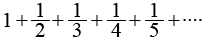# Python Example – Write a Python program to calculate the harmonic sum of n-1# (Python Example for Citizen Data Scientist & Business Analyst)

Write a Python program to calculate the harmonic sum of n-1.
Note: The harmonic sum is the sum of reciprocals of the positive integers.

Example:Sample Solution:

Python Code:

``````
def harmonic_sum(n):
if n < 2:
return 1
else:
return 1 / n + (harmonic_sum(n - 1))

print(harmonic_sum(7))
print(harmonic_sum(4))
``````

Sample Output:

```2.5928571428571425
2.083333333333333```

## Write a Python program to calculate the harmonic sum of n-1

#### Free Machine Learning & Data Science Coding Tutorials in Python & R for Beginners. Subscribe @ Western Australian Center for Applied Machine Learning & Data Science.```